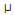# Electronics - Parallel Circuits

## Why Electronics Parallel Circuits?

In this section you can learn and practice Electronics Questions based on "Parallel Circuits" and improve your skills in order to face the interview, competitive examination and various entrance test (CAT, GATE, GRE, MAT, Bank Exam, Railway Exam etc.) with full confidence.

## Where can I get Electronics Parallel Circuits questions and answers with explanation?

IndiaBIX provides you lots of fully solved Electronics (Parallel Circuits) questions and answers with Explanation. Solved examples with detailed answer description, explanation are given and it would be easy to understand. All students, freshers can download Electronics Parallel Circuits quiz questions with answers as PDF files and eBooks.

## Where can I get Electronics Parallel Circuits Interview Questions and Answers (objective type, multiple choice)?

Here you can find objective type Electronics Parallel Circuits questions and answers for interview and entrance examination. Multiple choice and true or false type questions are also provided.

## How to solve Electronics Parallel Circuits problems?

You can easily solve all kind of Electronics questions based on Parallel Circuits by practicing the objective type exercises given below, also get shortcut methods to solve Electronics Parallel Circuits problems.

### Exercise :: Parallel Circuits - General Questions

1.

When parallel resistors are of three different values, which has the greatest power loss?

 A. The smallest resistance B. The largest resistance C. They have the same power loss. D. Voltage and resistance values are needed.

Explanation:

No answer description available for this question. Let us discuss.

2.

What is the product-over-sum result of 150 and 6800?

 A. 150 B. 146.7 C. 0.006 D. 6800

Explanation:

No answer description available for this question. Let us discuss.

3.

The voltage across any branch of a parallel circuit:

 A. varies as the total current varies B. is inversely proportional to total circuit resistance C. is equally applied to all branch conductances D. is dropped in proportion to each branch resistance

Explanation:

No answer description available for this question. Let us discuss.

4.

What is the total power loss if 2 kand 1 kparallel-connected resistors have an IT of 3 mA?

 A. 6W B. 36W C. 6 mW D. 36 mW

Explanation:

No answer description available for this question. Let us discuss.

5.

What happens to total resistance in a circuit with parallel resistors if one of them opens?

 A. It increases. B. It halves. C. It remains the same. D. It decreases.